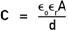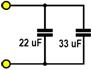Home / Electrical MCQs / Capacitors MCQs with Answers

Want create site? Find Free WordPress Themes and plugins.
1. A capacitor consists of two conducting surfaces called plates, separated by an insulating material called the:

 A. dielectric
 B. conductor
 C. electrode
 D. trielectric

2. Capacitance is the measure of the ability of a capacitor to:

 A. conduct a direct current
 B. hold an electric charge
 C. store current in a magnetic field
 D. repel dynamic eddy currents

3. A farad is the capacity of a capacitor that stores a charge of one coulomb at a potential difference of:

 B. one ampere
 C. one volt
 D. one ohm

4. A coulomb is the charge that passes a point in one second when a:

 A. voltage of one volt is generated
 B. couple of ohms are combined
 C. current does not flow in any direction
 D. current of one ampere flows

5. The charge on a capacitor can be determined using the formula:

 A. Q = VC
 B. Q = V/C
 C. Q = VC2
 D. Q = V2C

6. A 15 uF capacitor has been charged to a potential difference of 240 V. The charge on the capacitor will be:

 A. 3,600 C
 B. 0.003 6 C
 C. 0.0015 C
 D. 0.024 C

7. The capacitance of a capacitor varies according to three physical parameters. These are, the effective area of the plates, the distance between the plates and the:

 A. cross-sectional area of the plates
 B. supply voltage characteristic
 C. permittivity of the dielectric
 D. type of connecting lead used

8. For a capacitor consisting of two parallel plates, the capacitance can be found from the following equation:In the formula symbol ‘A’ stands for:

 A. cross-sectional distance between the plates
 B. distance between the plates in metres
 C. absolute permittivity of the dielectric
 D. area of the plates in square metres

9. The dielectric constant signifies the degree to which capacitance can be increased by replacing the:

 A. air between the plates with a dielectric
 B. air between the plates with a vacuum
 C. insulation between the plates with air
 D. vacuum between the plates with air

10. For a capacitor, the voltage per unit thickness necessary to cause breakdown is called the:

 A. dielectric constant of the capacitor
 B. dielectric strength of the insulating material
 C. capacitance of the insulating material
 D. electrostatic current limit of the capacitor

11. When capacitors are connected in series the total capacitance will be:

 A. more than the value of any one of the capacitors
 B. the same value as the largest one of the capacitors
 C. less than the value of any one of the capacitors
 D. the same value as the smallest one of the capacitors

12.
A 10 uF and a 22 uF capacitor have been connected in series as shown in the above diagram. The total resulting capacitance will be approximately:

 A. 32 uF
 B. 220 uF
 C. 2.2 uF
 D. 6.9 uF

13.
Two capacitors have been connected in series across a 24 V DC supply as shown. The voltage across the 33 uF capacitor will be:

 A. 14.1 V
 B. 9.9 V
 C. 7.9 V
 D. 11.3 V

14. Placing two or more capacitors in parallel has the same effect as:

 A. increasing the distance between the plates
 B. increasing the area of the plates
 C. increasing the dielectric strength of the capacitors
 D. decreasing the size of the connecting leads

15. Look at the following diagram:Two capacitors have been connected in parallel as shown. The total capacitance will be:

 A. 726 uF
 B. 72.6 uF
 C. 55 uF
 D. 13.2 uF

16. When a capacitor is connected to DC supply a charging current will flow. This current:

 A. flows through the capacitor’s electrostatic field
 B. will flow through the dielectric on each half cycle
 C. flows through the insulating material in the circuit
 D. does not flow through the capacitor

17. The time constant is the time taken for a capacitor to charge up to:

 A. 63.2 % of the applied voltage
 B. 86.4 % of the applied voltage
 C. 95.0 % of the applied voltage
 D. 99.3 % of the applied voltage

18.
With reference to the time constant curve shown above, the percentage of the supply voltage on a capacitor three time constants after charging has commenced will be:

 A. 86.4%
 B. 95.0%
 C. 98.2%
 D. 99.3%

19. Look at the following diagram:The time constant of the above circuit is:

 A. 4.0 mS
 B. 25 mS
 C. 2.5 S
 D. 4.0 S

20.
With reference to the above circuit, if the capacitor has been charged to 24 V, then the time taken to discharge to 15.16 V will be:

 A. 41.36 S
 B. 31.02 S
 C. 20.68 S
 D. 10.34 S

21.
With reference to the above circuit, if the capacitor is fully discharged and the switch connected to the charge position, the capacitor voltage will reach 20.74 V in:

 A. 20.68 S
 B. 41.36 S
 C. 19.34 S
 D. 47.0 S

22. The energy stored in the capacitor can be found using the formula:

 A. W = 0.5 C2V
 B. W = 0.5 CV2
 C. W = 0.5 CV
 D. W = 0.52 CV

23. A 47 uF capacitor has been charged from a 200 V DC supply. The energy stored in the capacitor will be:

 A. 1.88 J
 B. 0.94 J
 C. 4.25 J
 D. 8.14 J

24. Care must be taken when working with large value capacitors as they can:

 A. supply alternating current in an intermittent pattern
 B. change their value of capacitance when the supply is disconnected
 C. store high voltages for a long time after the supply is disconnected
 D. cause physical injury because of their extreme weight

25.
With reference to the above circuit, a high-value bleed-resistor has been permanently connected across capacitor C1 to:

 A. make sure the capacitor changes at a very fast rate
 B. keep the charge time of the capacitor to one time constant
 C. allow for the failure of the charging resistor R1 during switch-off
 D. ensure a controlled discharge when the supply is disconnected

26. If an oil-filled capacitor has less than its rated capacitance then the most likely cause would be that:

 A. some of the oil has leaked out
 B. the electrolyte has dried out
 C. it needs recharging on an AC supply
 D. the supply voltage has a DC component

27. To test a large capacitor, an ohm-meter is placed across the terminals of a known discharged capacitor. If there is capacity in the capacitor the resistance will be:

 A. high at first but decreasing
 B. low at first but increasing
 C. constant at all ranges of the ohm-meter
 D. 1000 ohms or less

28.
The capacitors shown are:

 A. oil filled
 B. electrolytic
 C. ceramic
 D. variable

Did you find apk for android? You can find new Free Android Games and apps.